Sie sind hier: ICP » R. Hilfer » Publikationen

# 4 Time Evolution of States

[210.2.1] In general, the set of observables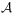of a physical system is not only a Banach space, but forms an algebra, more specifically, a C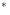-algebra . [210.2.2] In classical physics this algebra is commutative. [210.2.3] The states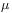of a physical system are normalized, positive linear functionals on its algebra of observables . [210.2.4] As such they are elements of the dual space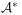. [210.2.5] The notation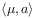is used for the value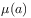of the observable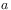in the state. [210.2.6] Convex combinations of states are again states. [210.2.7] If a state cannot be written as a convex combination of other states, it is called pure. [page 211, §0]    [211.0.1] Because the observable algebrais a subset of its bidual,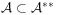, its elements can be considered as functions on the set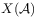of its charactersd (This is a footnote:) d A character is an algebraic *-homomorphism from a commutative C-algebra to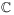., i.e.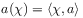for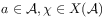. [211.0.2] By virtue of this correspondence, known as the Gelfand isomorphism [30, 31], a commutative C-algebra is isomorphic to the algebra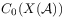of continuous functions on the setof its characters equipped with the weaktopology. [211.0.3] Characters are pure states.

[211.1.1] The time evolution of states is obtained from the time evolution of observables by passing to adjoints [28, 32]. [211.1.2]  The adjoint time evolution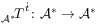with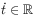consists of all adjoint operators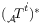on the dual space[33, 28]. [211.1.3] If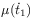denotes the state at time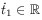, then equation (8) implies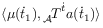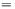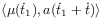(9)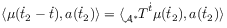where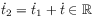is arbitrary. [211.1.4] For left translations the adjoint group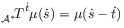(10)

is the group of right translations with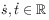. [211.1.5] The adjoint semigroup is weakcontinuous, but in general not strongly continuous, unless the Banach spaceis reflexive .